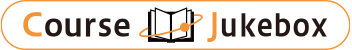### CourseDetailDegree
Master
Half
Course delivery methods
face-to-face
Subject
Mathematical sciences
Program
School
College of Social Engineering
Department
Campus
Main Campus
Classroom
Course Offering Year
Course Offering Month
September - January
Weekday and Period
Tuesday 2 Friday 34
Capacity
98
Credits
3
Language
English
Course Number
AM7006

### Applied Mathematics (Ⅰ) National Taiwan University

#### Course Overview

There are three chapters in this course. Chapter one covers the Cartesian Tensors, which are extensive used in the courses of Elasticity, Plasticity, Fluid mechanics, Piezoelasticity, and etc. Chapter two includes three parts. The first part introduces the existence and uniqueness theory for the 1st order ordinary differential equation (ODE) and 1st order system of ODE. The second part covers the solution of 1st order linear system of ODE, which is particular useful for the course of Dynamics. The third part of this chapter is designed to the solution of linear 2nd order ODE with unknown source functions. We introduces the concept of Dirac delta function, generalized functions, adjoint operators, Fredholm alternative theorem, Green�fs functions and modified Green�fs functions and the integral representation of the solution of 2nd order ODE. Finally, Chapter 3 also includes three parts. The 1st part introduces the classification of linear 2nd order PDE. The 2nd introduces the Green�fs function and the integral representation of solution of 2nd order linear PDEs. Free space Green�fs functions are solved first for infinite domain and then method of images are introduced for solving some simple finite domain PDE problems. The 3rd part introduces the eigenvalue problem of self-adjoint boundary value problems of 2nd order PDE, and the full/partial eigenfunction expansion for solving the linear 2nd order BVP or IBVP. Also included in this part are the Maximum-Minimum principle and unique theorems for Laplace/Poisson equation and Heat equation.

#### Learning Achievement

This course is aimed to let the graduate students own required knowledge in applied mathematics, which has applications in all aspects of mechanics, electricity and applied science.

#### Instructor

Mao Kuen Kuo,U Lei